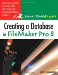# about calculations

FileMaker Pro supports two kinds of calculations: within individual records and across multiple records. Each is handled by a different field type.

Within-record calculations are accomplished using Calculation fields. A formula can combine other fields, constants, and built-in functions. For example, you could create a Calculation field that computes sales tax by multiplying an invoice total by a sales tax percentage (Total * Tax Percent) or by a constant (Total * .075). The computed sales tax would be different for each invoice. Calculations can be performed on Number, Text, Date, Time, or Container fields; the specified result must also be one of these data types. If you change the data in any field on which a calculation is based, the result is automatically recalculated. Unlike other field types, the result in a Calculation field cannot be edited. (You can copy the result, but you can't change it.)

Calculations that are computed across all or a subset of records in the database are performed using Summary fields. Although Summary fields can be placed in data entry layouts, it's more common to use them in reports (as we'll do in the Investment Minder reports described in Chapters 6 and 7).

The table below shows the names and formulas for two Calculation fields you'll create for the Main layout. Additional Calculation fields will be defined later; their formulas rely on fields in related tables that we haven't created yet.

Calculation Field Name

Formula

Bond_Current Value

Bond_Price + Bond_Interest

Stk_Total Cost

(Stk_Number of Shares * Stk_Price Per Share)+ Stk_Commission

Note: An asterisk (*) in a formula means to multiply.Creating a Database in FileMaker Pro 8: Visual QuickProject Guide
ISBN: 0321414837
EAN: 2147483647
Year: 2005
Pages: 115

Similar book on Amazon

flylib.com © 2008-2017.
If you may any questions please contact us: flylib@qtcs.net